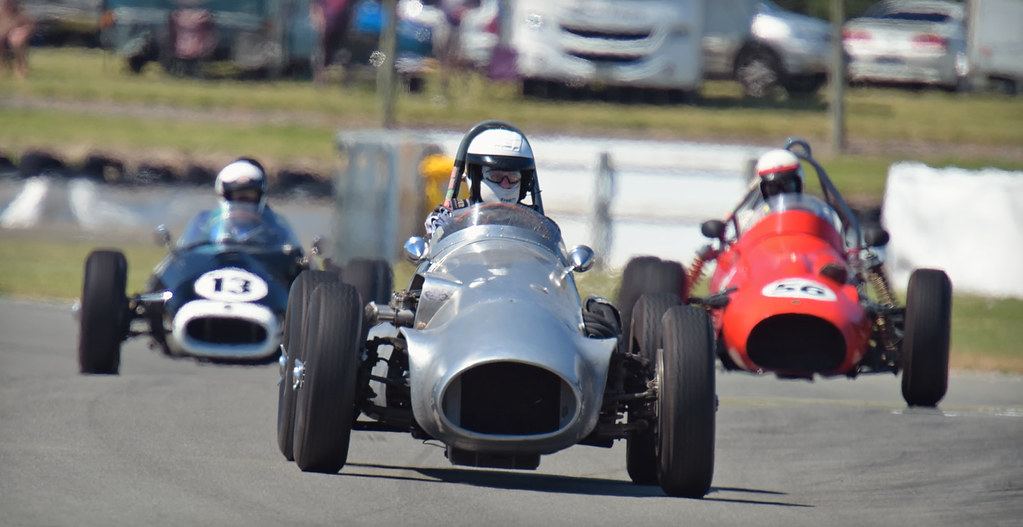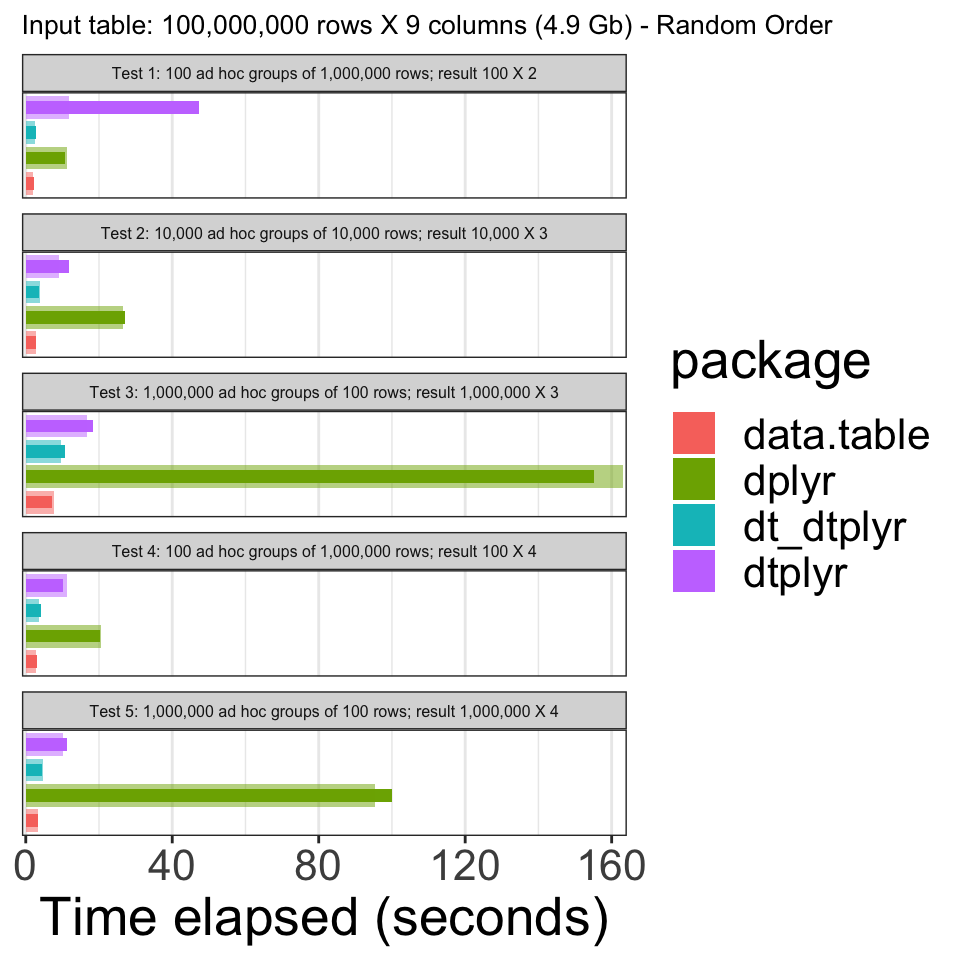# dtplyr speed benchmarksR has many great tools for data wrangling. Two of those are the dplyr and data.table packages. When people wonder which one should they learn it is often argued that dplyr is considerably slower compared with data.table.

Granted, data.table is blazing fast, but I personally find the syntax hard and un-intuitive and the speed difference doesn’t make much of a difference in most use cases I encountered.

The only frequent scenario where I’ve experienced a significant performance gap is when doing operations over a very large number of groups. This can happen when for example working with customer data, where each row describes a touch point or transaction and one is interested with calculating the number of rows per customer, monetary value of all transactions per customer etc.

Recently Rstudio released dtplyr package version 1.0.0 which provides a data.table backend for dplyr.

Using dtplyr requires learning almost no additional code. One initiates a data.table sequence using the `lazy_dt` function, after which regular dplyr code is written. Execution of the code is done only when calling `as_tibble` (or `as.data.frame` etc).

So for example a simple pipeline utilizing dtplyr for many group operations would look like:

``````mtcars %>%
lazy_dt() %>%
filter(wt < 5) %>%
mutate(l100k = 235.21 / mpg) %>% # liters / 100 km
group_by(cyl) %>%
summarise(l100k = mean(l100k))``````
``````## Source: local data table [?? x 2]
## Call:   `_DT1`[wt < 5][, `:=`(l100k = 235.21/mpg)][, .(l100k = mean(l100k)),
##     keyby = .(cyl)]
##
##     cyl l100k
##   <dbl> <dbl>
## 1     4  9.05
## 2     6 12.0
## 3     8 14.9
##
## # Use as.data.table()/as.data.frame()/as_tibble() to access results``````

The only caveat is, as with all other dbplyr like interfaces, that some of the more complex operations might not be supported.

Interestingly enough, I wasn’t able to find any bench-marking for dtplyr other than a walled piece on Medium. So I decided to go ahead and run a quick benchmark test myself.

In this post I’ll check by how much does dtplyr improve on dplyr and whether it’s performance is close enough to data.table to be considered a valid alternative.

To that end, I’ll reproduce some of the benchmarking done by data.table author Matt Dowle back at Dec 2018.

The bench-marking consist of:

• 5 simple queries: large groups and small groups on different columns of different types. Similar to what a data analyst might do in practice; i.e., various ad hoc aggregations as the data is explored and investigated.
• Each package is tested separately in its own fresh session. To that end I’ve restarted my machine before running the benchmark code for every package.
• Each query is repeated once more, immediately. This is to isolate cache effects and confirm the first timing. The first and second total elapsed times are plotted.

My analysis will diverge from the original in the following respects:

1. I’ll compare data.table, dtyplr and dplyr. I’ll also check how starting a dtplyr pipe with a `data.table` rather than a `data.frame` affects performance (dubbed dt_dtplyr below)
2. I’ll use my personal laptop instead of spinning up a virtual machine
3. I’m generating a much smaller dataset (~4.9 Gb). I think that is representative of some of the larger datasets I’ve worked with in-memory (for larger datasets I usually switch to Spark).

Other than that the code is mostly the same.

data.table benchmark code

``````require(data.table)
N <- 1e8
K <- 100
set.seed(1)
DT <- data.table(
id1 = sample(sprintf("id%03d", 1:K), N, TRUE), # large groups (char)
id2 = sample(sprintf("id%03d", 1:K), N, TRUE), # large groups (char)
id3 = sample(sprintf("id%010d", 1:(N / K)), N, TRUE), # small groups (char)
id4 = sample(K, N, TRUE), # large groups (int)
id5 = sample(K, N, TRUE), # large groups (int)
id6 = sample(N / K, N, TRUE), # small groups (int)
v1 = sample(5, N, TRUE), # int in range [1,5]
v2 = sample(5, N, TRUE), # int in range [1,5]
v3 = sample(round(runif(100, max = 100), 4), N, TRUE) # numeric e.g. 23.5749
)

q1a <- system.time(DT[, sum(v1), keyby = id1])
q1b <- system.time(DT[, sum(v1), keyby = id1])
q2a <- system.time(DT[, sum(v1), keyby = "id1,id2"])
q2b <- system.time(DT[, sum(v1), keyby = "id1,id2"])
q3a <- system.time(DT[, list(sum(v1), mean(v3)), keyby = id3])
q3b <- system.time(DT[, list(sum(v1), mean(v3)), keyby = id3])
q4a <- system.time(DT[, lapply(.SD, mean), keyby = id4, .SDcols = 7:9])
q4b <- system.time(DT[, lapply(.SD, mean), keyby = id4, .SDcols = 7:9])
q5a <- system.time(DT[, lapply(.SD, sum), keyby = id6, .SDcols = 7:9])
q5b <- system.time(DT[, lapply(.SD, sum), keyby = id6, .SDcols = 7:9])
data_table_results <- list(
q1a = q1a, q1b = q1b,
q2a = q2a, q2b = q2b,
q3a = q3a, q3b = q3b,
q4a = q4a, q4b = q4b,
q5a = q5a, q5b = q5b
)``````

dtplyr benchmark code

``````require(dplyr)
require(dtplyr)
N <- 1e8
K <- 100
set.seed(1)
DF <- data.frame(
stringsAsFactors = FALSE,
id1 = sample(sprintf("id%03d", 1:K), N, TRUE),
id2 = sample(sprintf("id%03d", 1:K), N, TRUE),
id3 = sample(sprintf("id%010d", 1:(N / K)), N, TRUE),
id4 = sample(K, N, TRUE),
id5 = sample(K, N, TRUE),
id6 = sample(N / K, N, TRUE),
v1 = sample(5, N, TRUE),
v2 = sample(5, N, TRUE),
v3 = sample(round(runif(100, max = 100), 4), N, TRUE)
)

q1a <- system.time(DF %>% lazy_dt() %>% group_by(id1) %>%
summarise(sum(v1)) %>% as_tibble())
q1b <- system.time(DF %>% lazy_dt() %>% group_by(id1) %>%
summarise(sum(v1)) %>% as_tibble())
q2a <- system.time(DF %>% lazy_dt() %>% group_by(id1, id2) %>%
summarise(sum(v1)) %>% as_tibble())
q2b <- system.time(DF %>% lazy_dt() %>% group_by(id1, id2) %>%
summarise(sum(v1)) %>% as_tibble())
q3a <- system.time(DF %>% lazy_dt() %>% group_by(id3) %>% summarise(sum(v1), mean(v3)) %>% as_tibble())
q3b <- system.time(DF %>% lazy_dt() %>% group_by(id3) %>%
summarise(sum(v1), mean(v3)) %>% as_tibble())
q4a <- system.time(DF %>% lazy_dt() %>% group_by(id4) %>%
summarise_at(vars(v1:v3), mean) %>% as_tibble())
q4b <- system.time(DF %>% lazy_dt() %>% group_by(id4) %>%
summarise_at(vars(v1:v3), mean) %>% as_tibble())
q5a <- system.time(DF %>% lazy_dt() %>% group_by(id6) %>%
summarise_at(vars(v1:v3), sum) %>% as_tibble())
q5b <- system.time(DF %>% lazy_dt() %>% group_by(id6) %>%
summarise_at(vars(v1:v3), sum) %>% as_tibble())

dtplyr_results <- list(
q1a = q1a, q1b = q1b,
q2a = q2a, q2b = q2b,
q3a = q3a, q3b = q3b,
q4a = q4a, q4b = q4b,
q5a = q5a, q5b = q5b
)``````

dt_dtplyr benchmark code

``````require(dplyr)
require(dtplyr)
library(data.table)
N <- 1e8
K <- 100
set.seed(1)
DF <- data.frame(
stringsAsFactors = FALSE,
id1 = sample(sprintf("id%03d", 1:K), N, TRUE),
id2 = sample(sprintf("id%03d", 1:K), N, TRUE),
id3 = sample(sprintf("id%010d", 1:(N / K)), N, TRUE),
id4 = sample(K, N, TRUE),
id5 = sample(K, N, TRUE),
id6 = sample(N / K, N, TRUE),
v1 = sample(5, N, TRUE),
v2 = sample(5, N, TRUE),
v3 = sample(round(runif(100, max = 100), 4), N, TRUE)
)

DF <- as.data.table(DF)

q1a <- system.time(DF %>% lazy_dt() %>% group_by(id1) %>%
summarise(sum(v1)) %>% as_tibble())
q1b <- system.time(DF %>% lazy_dt() %>% group_by(id1) %>%
summarise(sum(v1)) %>% as_tibble())
q2a <- system.time(DF %>% lazy_dt() %>% group_by(id1, id2) %>%
summarise(sum(v1)) %>% as_tibble())
q2b <- system.time(DF %>% lazy_dt() %>% group_by(id1, id2) %>%
summarise(sum(v1)) %>% as_tibble())
q3a <- system.time(DF %>% lazy_dt() %>% group_by(id3) %>% summarise(sum(v1), mean(v3)) %>% as_tibble())
q3b <- system.time(DF %>% lazy_dt() %>% group_by(id3) %>%
summarise(sum(v1), mean(v3)) %>% as_tibble())
q4a <- system.time(DF %>% lazy_dt() %>% group_by(id4) %>%
summarise_at(vars(v1:v3), mean) %>% as_tibble())
q4b <- system.time(DF %>% lazy_dt() %>% group_by(id4) %>%
summarise_at(vars(v1:v3), mean) %>% as_tibble())
q5a <- system.time(DF %>% lazy_dt() %>% group_by(id6) %>%
summarise_at(vars(v1:v3), sum) %>% as_tibble())
q5b <- system.time(DF %>% lazy_dt() %>% group_by(id6) %>%
summarise_at(vars(v1:v3), sum) %>% as_tibble())

dt_dtplyr_results <- list(
q1a = q1a, q1b = q1b,
q2a = q2a, q2b = q2b,
q3a = q3a, q3b = q3b,
q4a = q4a, q4b = q4b,
q5a = q5a, q5b = q5b
)``````

dplyr benchmark code

``````library(dplyr)
N <- 1e8
K <- 100
set.seed(1)
DF <- data.frame(
stringsAsFactors = FALSE,
id1 = sample(sprintf("id%03d", 1:K), N, TRUE),
id2 = sample(sprintf("id%03d", 1:K), N, TRUE),
id3 = sample(sprintf("id%010d", 1:(N / K)), N, TRUE),
id4 = sample(K, N, TRUE),
id5 = sample(K, N, TRUE),
id6 = sample(N / K, N, TRUE),
v1 = sample(5, N, TRUE),
v2 = sample(5, N, TRUE),
v3 = sample(round(runif(100, max = 100), 4), N, TRUE)
)

q1a <- system.time(DF %>% group_by(id1) %>% summarise(sum(v1)) %>% as_tibble())
q1b <- system.time(DF %>% group_by(id1) %>% summarise(sum(v1)) %>% as_tibble())
q2a <- system.time(DF %>% group_by(id1, id2) %>% summarise(sum(v1)) %>% as_tibble())
q2b <- system.time(DF %>% group_by(id1, id2) %>% summarise(sum(v1)) %>% as_tibble())
q3a <- system.time(DF %>% group_by(id3) %>% summarise(sum(v1), mean(v3)) %>% as_tibble())
q3b <- system.time(DF %>% group_by(id3) %>%
summarise(sum(v1), mean(v3)) %>% as_tibble())
q4a <- system.time(DF %>% group_by(id4) %>%
summarise_at(vars(v1:v3), mean) %>% as_tibble())
q4b <- system.time(DF %>% group_by(id4) %>%
summarise_at(vars(v1:v3), mean) %>% as_tibble())
q5a <- system.time(DF %>% group_by(id6) %>%
summarise_at(vars(v1:v3), sum) %>% as_tibble())
q5b <- system.time(DF %>% group_by(id6) %>%
summarise_at(vars(v1:v3), sum) %>% as_tibble())

dplyr_results <- list(
q1a = q1a, q1b = q1b,
q2a = q2a, q2b = q2b,
q3a = q3a, q3b = q3b,
q4a = q4a, q4b = q4b,
q5a = q5a, q5b = q5b
)``````We can see that using dtplyr improves the performance quite a bit, though still not as fast as data.table. It would seem however that most of the difference stems from the need to convert the `data.frame` object to a `data.table` one. That can be done once when reading in the file for example. Thus it would seem that ultimately the sacrifice in performance for the added benefit of tidy syntax (for those who dig tidy) isn’t too bad.

Personally, I’m hooked on the tidyverse and the dtplyr package is just another reason to keep using it, even for operations over a large number of groups.

## Session info

``sessionInfo()``
``````## R version 3.6.2 (2019-12-12)
## Platform: x86_64-apple-darwin15.6.0 (64-bit)
## Running under: macOS Mojave 10.14.6
##
## Matrix products: default
## BLAS:   /Library/Frameworks/R.framework/Versions/3.6/Resources/lib/libRblas.0.dylib
## LAPACK: /Library/Frameworks/R.framework/Versions/3.6/Resources/lib/libRlapack.dylib
##
## locale:
##  en_US.UTF-8/en_US.UTF-8/en_US.UTF-8/C/en_US.UTF-8/en_US.UTF-8
##
## attached base packages:
##  stats     graphics  grDevices utils     datasets  methods   base
##
## other attached packages:
##   data.table_1.12.8 pander_0.6.3      dtplyr_1.0.0      forcats_0.4.0
##   stringr_1.4.0     dplyr_0.8.5       purrr_0.3.4       readr_1.3.1
##   tidyr_1.0.3       tibble_3.0.1      ggplot2_3.3.0     tidyverse_1.3.0
##  pacman_0.5.1
##
## loaded via a namespace (and not attached):
##   tidyselect_1.1.0 xfun_0.12        haven_2.2.0      lattice_0.20-38
##   colorspace_1.4-1 vctrs_0.3.0      generics_0.0.2   htmltools_0.4.0
##   yaml_2.2.1       utf8_1.1.4       rlang_0.4.6      pillar_1.4.4
##  glue_1.4.1       withr_2.1.2      DBI_1.1.0        dbplyr_1.4.2
##  modelr_0.1.5     readxl_1.3.1     lifecycle_0.2.0  munsell_0.5.0
##  blogdown_0.17    gtable_0.3.0     cellranger_1.1.0 rvest_0.3.5
##  evaluate_0.14    labeling_0.3     knitr_1.27       fansi_0.4.1
##  broom_0.5.3      Rcpp_1.0.4.6     scales_1.1.0     backports_1.1.5
##  jsonlite_1.6.1   farver_2.0.3     fs_1.4.1         hms_0.5.3
##  digest_0.6.25    stringi_1.4.6    bookdown_0.17    grid_3.6.2
##  cli_2.0.2        tools_3.6.2      magrittr_1.5     crayon_1.3.4
##  pkgconfig_2.0.3  ellipsis_0.3.1   xml2_1.2.2       reprex_0.3.0
##  lubridate_1.7.8  assertthat_0.2.1 rmarkdown_2.0    httr_1.4.1
##  rstudioapi_0.11  R6_2.4.1         nlme_3.1-143     compiler_3.6.2``````